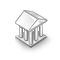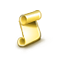# Statistics.mqh 函数 - MetaTrader 5程序库

1592

2014.01.28 06:50

2016.11.22 07:33

Mediana 中位数计算
Mediana50 50％四分位范围的中位数计算
Average 样本算术平均值计算
Average50 50％四分位范围的样本算术平均值计算
SweepCenter 中置计算
AverageOfEvaluations 计算五个上部评估的平均值
Variance 样本方差计算
ThirdCentralMoment 三阶中心矩计算
FourthCentralMoment 四阶中心矩计算
Asymmetry 样本非对称计算
Excess 样本超额计算
Excess2 另一种样本超额计算方法
Gamma Euler 的伽玛函数计算, x>0.
GammaStirling Euler 的伽玛函数值计算, 对于 x>33 (Stirling 的近似)
VarianceOfSampleVariance 计算样本方差的方差
VarianceOfStandartDeviation 计算标准方差的方差
VarianceOfAsymmetry 非对称样本方差计算
VarianceOfExcess 样本超额方差计算
VarianceOfAverage 样本均值方差计算
Log 对数计算
CensorCoeff 终检率计算
HistogramLength 计算直方图柱线的优化值
Resize 计算直方图的数组元素的优化值
Histogram 创建直方图至 *.csv 文件
Cov 样本协方差计算
Corr 样本相关计算
VarianceOfCorr 相关样本方差计算
AutoCorr 自相关计算
AutoCorrFunc 自相关函数的计算
aCoeff 计算线性回归方程 a 的解 (y=a*x+b)
bCoeff 计算线性回归方程 b 的解 (y=a*x+b)
LineRegresErrors 计算线性回归误差
eVariance 计算线性回归误差方差
aVariance 计算线性回归 a 参数的方差
bVariance 计算线性回归 b 参数的方差
DeterminationCoeff 确定解计算
ArraySeparate 切分 arr[n] 数组至两个数组
ArrayUnion 合并两个数组至 arr[n] 类型
WriteArray 写一维数组至 *.csv 文件
WriteArray2 写二维数组至 *.csv 文件

```//+------------------------------------------------------------------+
//|                                                         test.mq5 |
//|                        Copyright 2012, MetaQuotes Software Corp. |
//|                                              http://www.mql5.com |
//+------------------------------------------------------------------+
#property version   "1.00"

#include <Statistics.mqh>
//+------------------------------------------------------------------+
//| Script program start function                                    |
//+------------------------------------------------------------------+
void OnStart()
{
//--- 指定两个样本值.
double arrX={3,4,5,2,3,4,5,6,4,7};
double arrY={7,4,1,2,1,6,9,2,1,5};
//--- 计算平均值
double mx=Average(arrX);
double my=Average(arrY);
//--- 使用平均值计算方差
double dx = Variance(arrX,mx);
double dy = Variance(arrY,my);
//--- 非对称值和超额
double as=Asymmetry(arrX,mx,dx);
double exc=Excess(arrX,mx,dx);
//--- 协方差和相关值
double cov=Cov(arrX,arrY,mx,my);
double corr=Corr(cov,dx,dy);
//--- 在日志文件中输出值
PrintFormat("mx=%.6e",mx);
PrintFormat("my=%.6e",my);
PrintFormat("dx=%.6e",dx);
PrintFormat("dy=%.6e",dy);
PrintFormat("As=%.6e",as);
PrintFormat("exc=%.6e",exc);
PrintFormat("cov=%.6e",cov);
PrintFormat("corr=%.6e",corr);
}```

`double dx = Variance(arrX,mx);`EAX_Mysql - MySQL library价格在布林通道对象描述激活器趋势百分比